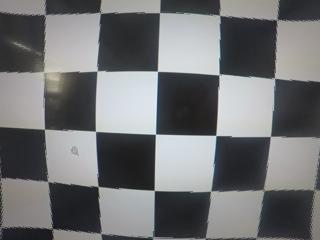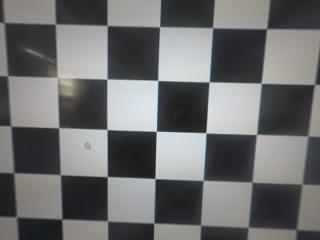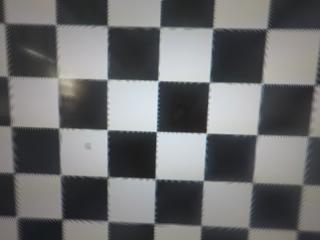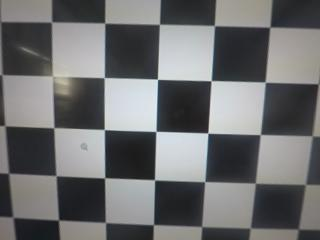In recent less apocalyptic times, I used to play dodgeball - a team sport involving throwing foam balls at members of the opposing team. I hope that the COVID-19 outbreak passes soon and I can play it again, but in the meantime the closest I can get is watching videos of it and doing image processing on them. Our team records our matches for later analysis using a GoPro camera. The game is played in a rectangular court surounded by a net, and we mount the camera in the corner of the net so that the net does not obstruct the camera’s view of the court, and the camera’s field of view includes the entire court.

There are a number of issues with the recorded video which I wanted to fix:

• The lens is a fisheye lens, and the captured video contains barrel distortion. This is not good for watching balls being thrown, because it makes it difficult to see if the trajectory of the ball was a straight line, or a curve.
• The net that the camera is attached to is somewhat flexible and is frequently hit by balls and players. This causes the camera to shake violently, which makes for shaky videos.

For this post, I’ll focus on just the first issue of lens distortion.

I decided to make a first attempt using FFMpeg. FFMpeg is easy to get started with since it has a CLI and doesn’t require writing any code. It can read and write basically any media format, and also has a selection of filters that can be used to transform videos, some of which seemed relevant to my task. It’s also open source, which means it’s yours to do what you want with.

To test the various filters, I took a picture of the OpenCV chessboard calibration pattern, which looks like this:

The photo of the pattern (which was on a TV screen, which is flat), looks like this:

If you didn’t know that the test image was a chess board, it wouldn’t be obvious that in real life its all straight lines and right angles. My aim is to take this image from the camera, and produce an output that looks (geometrically) like the test image.

## lenscorrection filter

The lenscorrection filter warps the image to correct for lens distortion according to the supplied parameters appropriate for the camera and lens. It acceps two parameters $k_1$and $k_2$, which correspond to a quadratic and cubic correction factor applied to the radius of a pixel from the center of the image.

### Stack overflow

Obviously I’m not the first person to have this problem, and consequently I found a stack overflow thread where other people had posted various values for the same and slightly different cameras. Most of these did not work well at all, but one of them worked somewhat:

$ffmpeg -i chess-raw.png -vf lenscorrection=k1=-0.227:k2=-0.022 chess-lenscorrection-so.pngchessboard corrected with lenscorrection filter using parameters from stack overflow You could reasonably say that this is much worse than the raw image, even though in a sense it is closer to the ideal. ### Hugin I tried to use the Hugin lens calibration tool to find suitable values of $k_1$ and $k_2$. The model of lens distortion that the lenscorrection filter uses is called poly5, and the value of $r_d$ (the radius in the original distorted image) is given as a function of $r_u$ (the radius in the corrected output image) as follows: $r_d=r_u(1+k_1r_u^2+k_2r_u^4)$ Meanwhile, Hugin uses the following model (which is called ptlens): $r_d=r_u⋅(ar_u^3+br_u^2+cr_u+1−a−b−c)$ To try to find common ground between these two models, we need to dispense with $k_2$, because there is no $r_u^5$ term in ptlens. Similarly $a$ and $c$ have to go, because there is no $r_u^2$ or $r_u^4$ term in poly5. So setting $k_2=a=c=0$ the two equations simplify to the following: $r_d=r_u(1+k_1r_u^2)$ $r_d=r_u⋅(1+br_u^2−b)$ If $b=k_1$, these equations are “almost” the same. Unfortunately I couldn’t get rid of the $br_u$ term, but this is the closest thing I could find to an equivalence between the two models. Presumeably, the mismatched linear term would simply scale the image. So I took some pictures of apartment blocks and asked Hugin to find a ptlens model using only $b$. Hugin gave the value $-0.08101$, so I used that value as $k_1$ in the lenscorrection filter. This was the result: $ ffmpeg -i chess-raw.png -vf lenscorrection=k1=-0.08101:k2=0 chess-lenscorrection-hugin.png


Obviously, this didn’t work well at all. I’m not sure where I went wrong.

This filter is simple and reasonably fast, but I could not find values for $k1$ and $k2$ which did a particularly good job of correcting for the distortion on my camera. Also, it only performs nearest neighbour interpolation, which results in visible aliasing in the output (I resized the images to 320x240 so that this is obvious).

## lensfun filter

The lensfun filter is a wrapper for the lensfun library, which performs correction for many types of lens distortion including barrel distortion. It also includes a database of cameras and lenses and their measured characteristics. I found that the latest development version had a database entry for the GoPro HERO5 Black camera that I was using.

After some experimentation, I worked out that the parameters in the database were appropriate only for certain camera settings. The camera has a “Field of View” setting, with 3 different settings using a fisheye projection (more specifically, an imperfect stereographic projection, as I learned from the lensfun database), and also a “linear” setting (which results in standard rectilinear projection, but with a much smaller field of view). The camera also has a video stabilisation feature which results in a 10% crop of the recorded video (although the stabilisation itself did not work well). I found that selecting the “Wide” setting and turning off the stabilisation resulted in a video that was correctly undistorted by lensfun using the parameters in the database.

$ffmpeg -i chess-raw.png -vf 'lensfun=make=GoPro:model=HERO5 Black:lens_model=fixed lens:mode=geometry:target_geometry=rectilinear' chess-lensfun.pngchessboard corrected with lensfun ### scale parameter Correcting for geometric lens distortion is a process that warps the image - i.e. it “moves” pixels from the source image to a different location in the destination image - or put another way, it maps pixels in the destination image to a different point in the source image. This means that the rectangular source image will not necessarily be mapped to a rectangle in the destination image. So there is a compromise to be made when choosing the scale of the output - either the output can be rectangular and have no blank areas (at the expense of discarding some of the input image), or it can include the entire input image (at the expense of having some blank areas in the output). Lensfun has a parameter called scale which controls this compromise. Unfortunately the FFMpeg filter wrapping lensfun did not have such an option. So I made a patch to add the option and pass it through to lensfun. This patch has been applied (hooray), so the following now works: $ ffmpeg -i chess-raw.png -vf 'lensfun=make=GoPro:model=HERO5 Black:lens_model=fixed lens:mode=geometry:target_geometry=rectilinear:scale=0.4' chess-lensfun-scaled.png


### interpolation

The default interpolation is bilinear, which is acceptable, and looks much better than the nearest neighbour interpolation as used in the lenscorrection filter. But lensfun also supports lanczos interpolation, which in theory should be better. I suspect there is a bug in it though, because the result doesn’t look as good as the default:

$ffmpeg -i chess-raw.png -vf 'lensfun=make=GoPro:model=HERO5 Black:lens_model=fixed lens:mode=geometry:target_geometry=rectilinear:interpolation=lanczos' chess-lensfun-lanczos.pngchessboard corrected with lensfun, using lanczos interpolation The lensfun filter is significantly slower than the lenscorrection filter, but did a much better job (more accurate corrections, and better interpolation). It also provides the ability to choose from multiple projections for the output (e.g. correct for the imperfections in the lens but maintain the stereographic projection, or output a equirectangular projection instead, etc), which I found interesting. ## Aside: interesting learnings about lens distortion and projections The term “distortion” comes with a negative connotation, but there are many reasonable ways to project a view of a 3D world onto a 2D image, each with different compromises. These projections are mappings from the angle at which light enters the camera lens $\theta$ (relative to the direction the lens is facing), to a distance $r$ (r for radius) from the center of the image. For example: • rectilinear projection $r=tan(\theta)$: straight lines in the world appear straight in the image, but areas far from the center are stretched alot, and it is impossible to diplay points 90 degrees or more from the center (i.e. the image cannot show what is directly to the side or behind the camera). Most images use this projection, and most image processing algorithms assume that it is used. • stereographic projection $r=2tan(\theta/2)$ (approximately what the GoPro HERO5 Black produces with the “wide” FoV setting): Maintains angles as seen from the lens, does not stretch the edges of the image as much as the rectilinear projection, and works for any angle under 180 degrees (i.e. any direction except directly backwards). Does not maintain straight lines. There are many other projections - see the lensfun list of projections and Wikipedia’s fisheye lens article. Similarly, there are many different models for correcting the projection produced by real cameras and lenses (which may not be a simple mathematical formula) to suit one of the standard projections. These are usually polynomials applied to the radius of a pixel. Lensfun supports 4 different models for example. The lenscorrection filter appears to use the same model as lensfun’s LF_DIST_MODEL_POLY5. The lensfun database entry for my camera uses the different LF_DIST_MODEL_POLY3 model. Lensfun makes a relatively small correction to convert the image to a standard stereographic projection before separately converting it to the rectilinear projection. ## v360 filter After I did most of these experiments, the v360 filter was added to ffmpeg, which is very exciting. Like lensfun, it can convert between various common projections. Unlike lensfun, it does not do polynomial corrections to account for real world differences from standard projections, and it does not have a database of cameras and lenses. Instead, there are parameters to specify the standard projection and field of view of the input, and of the output. I found that the GoPro website helpfully lists the horizontal, diagonal, and vertical field of view for each of the field of view settings on my camera, and I know from reading the lensfun database that my camera creates images that are closest to a stereographic projection. $ ffmpeg -i chess-raw.png -vf 'v360=input=sg:ih_fov=122.6:iv_fov=94.4:output=flat:d_fov=149.2:pitch=-90:w=320:h=240' chess-v360.png


This is not perfect (since my camera does not produce a perfect stereographic projection), but in my opinion it doesn’t look too bad. The curviness is less noticeable in the central area of the image, so if you adjust the output field of view enough that there are no unmapped areas, it looks better:

$ffmpeg -i chess-raw.png -vf 'v360=input=sg:ih_fov=122.6:iv_fov=94.4:output=flat:d_fov=121:pitch=-90:w=320:h=240' chess-v360-zoom.pngchessboard corrected with v360, scaled to fill entire output Roughly, v360 works in three stages. Firstly, it maps each input pixel to a vector, which represents the direction where the light came from (this is the inverse of the input projection). Then it optionally changes the camera angle according to the yaw/pitch/roll options (i.e. the direction vector for each pixel is rotated equally). This is different from cropping/translating the projected image because it moves the center of the image which all the projections are relative to. As a result, the resulting projected image looks exactly like it would have looked if the camera was facing in a different direction. The final step is to map these vectors to the destination image according to the chosen output projection and field of view. Here’s an example of using the rotation parameters to turn the virtual camera downwards by 15 degrees: $ ffmpeg -i chess-raw.png -vf 'v360=input=sg:ih_fov=122.6:iv_fov=94.4:output=flat:d_fov=149.2:pitch=-105:w=320:h=240' chess-v360-down.png


When I originally wrote this post, I found a few small but annoying bugs in the filter, which have since been fixed:

• Although it can deduce the horizontal/vertical field of view if only the diagonal field of view is privided, it did not correctly do this unless the input projection was “fisheye” or “flat” (rectilinear), because that code was not implemented at the time. I worked around this problem by directly providing the horizontal and vertical field of view as parameters.
• The stereographic projection had a built in pitch of 90 degrees, so if the input was stereographic, one had to use a pitch of -90 degrees to prevent the virtual camera from facing upwards instead of forwards.
• The yaw and roll options both seemed to perform roll (i.e. twisting the camera lens) - while yaw was impossible (turning the camera sideways).

I also found some limitations (which remain):

• The default output image dimensions are not sane.
• The scale of the output is determined but the output field of view, so it is up to you to determine what that should be.

Despite not performing polynomial corrections and not having a database of lenses, v360 has a few advantages over lensfun. It is much faster, perhaps due to the presence of a SIMD optimised implementation in assembly. The rotations are useful if the camera wasn’t facing quite the right way, and produce much better output in this case than cropping. The lanczos interpolation works well. Its scope is smaller than lensfun and the code in my opinion is easier to read, if you like doing that.

## Speed, quality, features: pick 2

If you don’t care about speed, you could use both lensfun (to perform accurate correction for a particular real world lens), and v360 (to use its perspective rotation feature):

\$ ffmpeg -i chess-raw.png -vf 'lensfun=make=GoPro:model=HERO5 Black:lens_model=fixed lens:mode=geometry:target_geometry=fisheye_stereographic,v360=input=sg:ih_fov=122.6:iv_fov=94.4:output=flat:d_fov=140:pitch=-105:w=320:h=240' chess-lensfun-v360.png


## Conclusion

For my use case, I’ve found that using v360 is the best compromise. My camera produces images that are close enough to the stereographic projection that if I convert them to rectilinear using v360 they appear straight, at least if you aren’t thinking about lens distortion. The perspective rotation feature is useful if the camera wasn’t quite level, the interpolation works well, and it is faster than lensfun. The right compromise depends on your needs.

In the future, I might write a similar post about video stabilisation. I’m also currently working on a project using libavcodec, OpenCL, and OpenCV that I hope will be capable of video decoding, lens correction, stabilisation, and reencoding all on the GPU, which should be much faster than all these methods which run on the CPU.